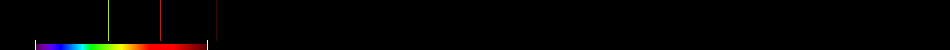# Information for Sensor and Index

## Formula

General Formula $\left(1.5\frac{2.5\left({\mathrm{800nm}}-{\mathrm{670nm}}\right)-1.3\left({\mathrm{800nm}}-{\mathrm{550nm}}\right)}{\sqrt{{\left(2{\mathrm{800nm}}+1\right)}^{2}-\left(6{\mathrm{800nm}}-5\sqrt{{\mathrm{670nm}}}\right)-0.5}}\right)$ $\left(1.5\frac{2.5\left({\mathrm{infrared}}-{\mathrm{red}}\right)-1.3\left({\mathrm{infrared}}-{\mathrm{green}}\right)}{\sqrt{{\left(2{\mathrm{infrared}}+1\right)}^{2}-\left(6{\mathrm{infrared}}-5\sqrt{{\mathrm{red}}}\right)-0.5}}\right)$ Automatic

## Sensor

Name CBERS-1/CBERS-2 8 450-890 2.7-260 0 Brazil and China 2003-10-21 CBERS-2 is identical to CBERS-1 and was launched 2003-10-21 yes

## Index

Name Modified Chlorophyll Absorption in Reflectance Index 2 MCARI2 $\left(1.5\frac{2.5\left({\mathrm{800nm}}-{\mathrm{670nm}}\right)-1.3\left({\mathrm{800nm}}-{\mathrm{550nm}}\right)}{\sqrt{{\left(2{\mathrm{800nm}}+1\right)}^{2}-\left(6{\mathrm{800nm}}-5\sqrt{{\mathrm{670nm}}}\right)-0.5}}\right)$ 550,670,800 Original formula

## References